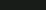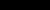# ResearchI am a mathematician, as well as a theoretical physicist, researching mathematical structures of quantum field theory (QFT). Primarily I am working in the framework of the algebraic quantum field theory (AQFT). Although my main goal is to understand the non-perturbative structure of QFT, recently I got interested also in the perturbative approach (pAQFT). My main research topics are:
1. Physics:
• algebraic quantum field theory
• renormalization
• quantum field theory on curved spacetimes
• Batalin-Vilkovisky formalism
• quantum gravity
• noncommutative geometry
2. Mathematics:
• operator algebras
• functional analysis
• infinite dimensional calculus and differential geometry
• infinite dimensional Lie groups
• homological methods in physics
• noncommutative geometry